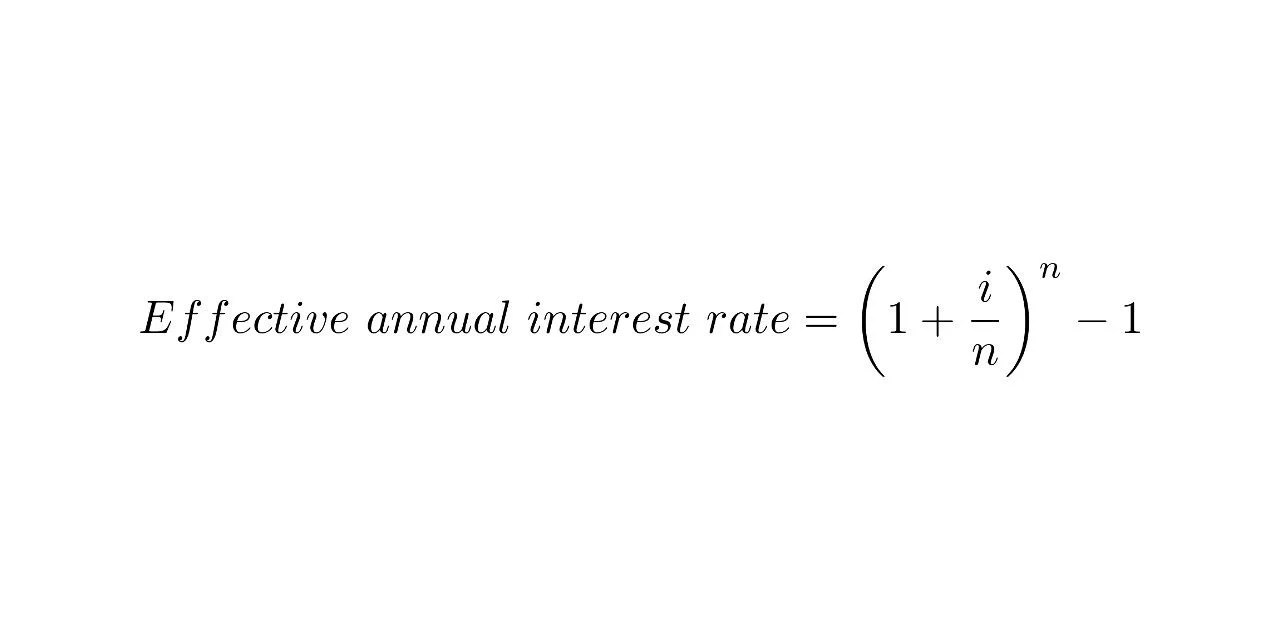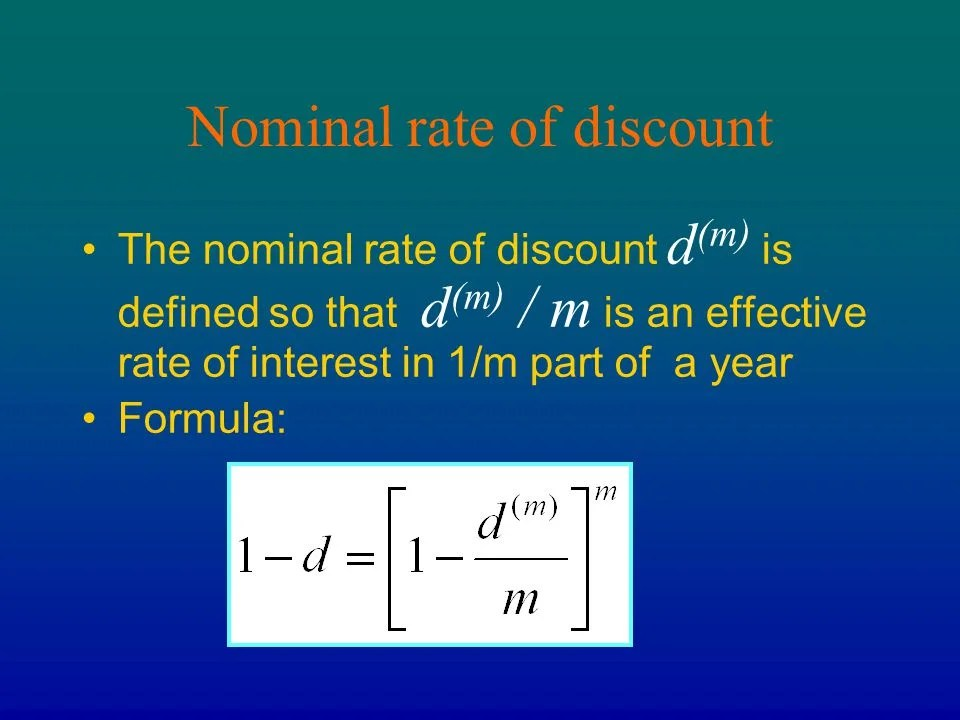# Effective and Nominal Rate of discount

14th February 2020

The effective annual interest rate is the interest rate that is actually earned or paid on an investment, loan or other financial product due to the result of compounding over a given time period. It is also called the effective interest rate, the effective rate or the annual equivalent rate.#### Nominal Rate of discount

Nominal interest rate refers to the interest rate before taking inflation into account. Nominal can also refer to the advertised or stated interest rate on a loan, without taking into account any fees or compounding of interest.

Nominal interest rates exist in contrast to real interest rates and effective interest rates. Real interest rates tend to be important to investors and lenders, while effective rates are significant for borrowers as well as investors and lenders.

Unlike the nominal rate, the real interest rate takes the inflation rate into account. The equation that links nominal and real interest rates can be approximated as nominal rate = real interest rate + inflation rate, or nominal rate – inflation rate = real rate.

To avoid purchasing power erosion through inflation, investors consider the real interest rate, rather than the nominal rate. One way to estimate the real rate of return in the United States is to observe the interest rates on Treasury Inflation-Protected Securities (TIPS). The difference between the yield on a Treasury bond and the yield on TIPS of the same maturity provides an estimate of inflation expectations in the economy.The nominal rate of discount d(m) is defined so that d(m) / m is an effective rate of interest in 1/m part of a year. Formula: